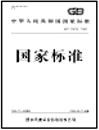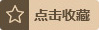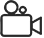# ASTM C623:2021现行Standard Test Method for Young's Modulus, Shear Modulus, and Poisson's Ratio for Glass and Glass-Ceramics by ResonanceThis test method covers the determination of the elastic properties of glass and glass-ceramic materials. Specimens of these materials possess specific mechanical resonance frequencies which are defined by the elastic moduli, density, and geometry of the test specimen. Therefore the elastic properties of a material can be computed if the geometry, density, and mechanical resonance frequencies of a suitable test specimen of that material can be measured. Young's modulus is determined using the resonance frequency in the flexural mode of vibration. The shear modulus, or modulus of rigidity, is found using torsional resonance vibrations. Young's modulus and shear modulus are used to compute Poisson's ratio, the factor of lateral contraction.